# Archeops Data Products on LAMBDA

### CMB Power Spectrum

These two files (in ASCII and FITS versions) contain the Archeops power spectrum for each of 25 bins. The table contains 6 columns: l_min, l_max, l(l+1)C_l/2pi, total error, statistical error, cosmic variance. Units are uK^2.

M. Tristram and J.F. Macias-Perez - November 2004

### CMB Covariance Matrix

These two files (in ASCII and FITS versions) contain the covariance matrix for the Archeops power spectrum (in uK^4). It is a 25x25 matrix describing the 25 bins of the released power spectrum. The error bars for each bin can be computed from the square root of the diagonal of the covariance matrix.

M. Tristram and J.F. Macias-Perez - November 2004

### CMB Window Functions

These two files contain the window functions (in ASCII and FITS versions) for the Archeops power spectrum bins. The integral of the window functions are normalized to 1 for each of the 25 bins and are computed between l=0 and l=1000. The table contains a float array with dimension 25 x 1001 (25 columns by 1000 rows). The window functions are defined as follows (with implicit sum over repeated indices):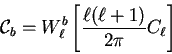(1)

In the MASTER framework (Hivon et al., 2002), if we define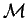as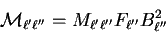(2)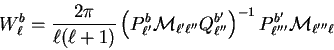(3)

Where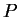and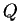are the binning and reciprocal operators.

Using this definition, we have for each bin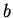: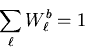(4)

M. Tristram and J.F. Macias-Perez - November 2004

### Dust Polarization Power Spectra

Dust polarized angular power spectra as measured by Archeops at 353 GHz for different galactic cuts (5, 10 and 20 deg). Tables contains : l_min, l_max, l(l+1)C_l^TT/(2 Pi) error, (l+1)C_l^TE/(2 Pi) error, (l+1)C_l^EE/(2 Pi) error, (l+1)C_l^BB/(2 Pi) error Units ARE microK_RJ^2.

Go To Archeops Product Page

A service of the HEASARC and of the Astrophysics Science Division at NASA/GSFCHEASARC Director: Dr. Andrew F. Ptak
LAMBDA Director: Dr. Thomas M. Essinger-Hileman
NASA Official: Dr. Thomas M. Essinger-Hileman
Web Curator: Mr. Michael R. Greason